# Effects of system topologies’ attributes on average consensus algorithm - part I.

Autor: M. Kenyeres, T. Danhel, J. Kenyeres, V. Škorpil <kenyeres(at)phd.feec.vutbr.cz>, Pracoviště: Vysoké učení technické v Brně, Téma: Aplikace, sítě a služby, Vydáno dne: 23. 06. 2015

In this publication, we focused our attention on examining the effects of the topology on the average consensus algorithm. We examined the number of the necessary iterations for a system to achieve the consensus while altering several parameters: the speed of the algoritm convergence (ε), dispersion and shift of the initial values. In our analysis, we considered networkspokracovanie with the tree, ring, star and mesh topology. At the end, we compared the obtained results.

Keywords: distributed computing; average consensus; ring/star/tree/mesh topologies

### Introduction

This paper deals with the effect of the topologies on the number of the necessary iterations for a system to achieve the consensus when the speed of the algoritm convergence (ε) changed. We executed five experiments on four well-known topologies: tree, ring, star and mesh. These topologies differ from each other in aspects as such the average conectivity, the maximal distance in hops, the relative connectivity, the connectivity of the best connected entity etc. Each of the examined topologies is characterized by a specific set of the features.

Tree topology

The examined tree topology is shown in Fig.1. It consists of 32 elements with different connectivity. There are 17 entities connected to one adjacent neighbor and 15 with the connectivity equals to 3. The maximal distance is 8 hops and the most connected entity has 3 adjacent neighbors and so, this topology is predestined to have a wide interval of the convergence.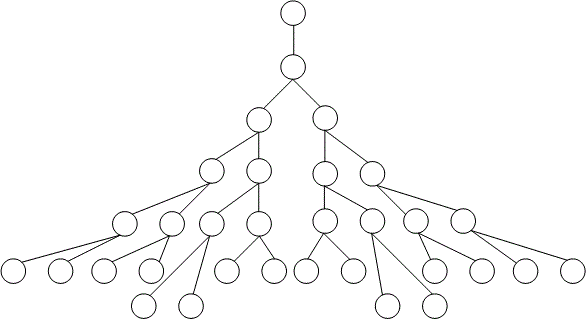Fig. 1 Tree topology

Ring topology

The ring topology of the size of 32 entities is shown in Fig.2. It is formed by entities whose connectivities do not differ from each other and equal to 2. The highest distance between two entities is 16 hops. Since the most connected entity has 2 adjacent neighbors, this topology is assumed to have the widest convergence interval within all the examined topologies in this paper.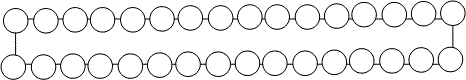Fig. 2 Ring topology

Star topology

The star topology is shown in Fig.3. Its size is 32 entities just like in the previous topologies. The topology contains one entity connected to all the others and whose connectivity is 31 adjacent neighbors. Other entities are connected just to this central one and therefore, their connectivities are just one neighbor. The maximal hop distance is 2 and the maximal connectivity equals to 31.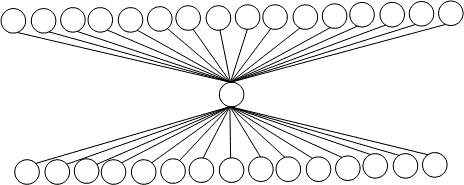Fig. 3 Star topology

Mesh topology

As depicting the mesh topology would not be transparent, we have decided not to show this topology. We considered a fully-connected mesh whose size is 32 entities. All the entities are connected to each other and so, the maximal hop distance is 1 and the maximal connectivity is 31. Thus, this topology is predestined to reach the consensus fastest.

### Average consensus

The distributed algorithms’ functionality relates to parallel distributed systems formed by separated subparts whose relative communication is very limited . Nevertheless, the entities forming a distributed system are able to cooperate with one another and fulfil a specific functionality. In this paper, we focused on average consensus algorithm and considered a distributed system executing it. Average consensus is a distributed consensus algorithm whose output is represented by the average value calculated from the initial values of all the entities in a system. This state is achieved in such a way that each entity iteratively converges to the average by updating its inner state according to the inner state from the previous iteration and the information provided by the adjacent neighbors. In order to describe a distributed system, it is necessary to define a set of mathematical tool to describe its behaviour , . As first, we defined a two-dimensional field in which we insert entities' values at every iteration.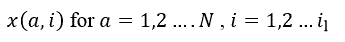(1)

Here, N is the number of the entities in a system, a is the identity number of a particular entity and i labels the number of an iteration. The parameter il labels the last iteration and also determines the number of iterations required for a system to reach the consensus. We assume that the algorithm is executed in a system which can be described by a graph structure. Therefore, we define the adjacent field A(a,b) determining the neighborhood relations between entities ,. It is a diagonally symmetric field of a square shape and its size is determined by N. Its elements equal to 1 or 0. If two entities are each other’s neighbor, then(2)

In the second case, if they are not or a = b, the following statement is valid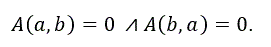(3)

As the example, consider the system shown in Fig.4.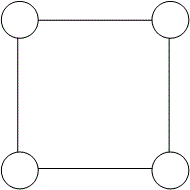Example topology

Then we can create the adjacent field by applying the conditions (2) and (3) and show it in Fig.5.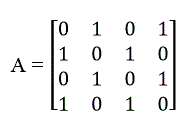Adjacency matrix of the example topology

Each entity is assigned the identity number which allows it to calculate its initial value. The identity number is set deterministically and is unique for each entity . We assume that each entity's initial value is either equaled to the identity number or determined according to formula (4) or (5).(4)

or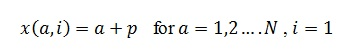(5)

Here, the parameter r determines a dispersion of the initial values and p is the parameter to express an initial shift. We defined the initial values this way to demonstrate the relation between initial values and a system's topology. As mentioned, the entities forming a system executing the average consensus reach the consensus in an iterative distributed way. The final value which they achieve is defined as follows :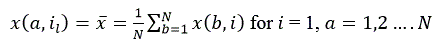(6)

The iterative distributive computing means that every element update its inner value at each iteration according to the following formula:(7)

Here, ε is the parameter determining the speed of the algoritm's convergence (also the convergence interval) and is defined as follows :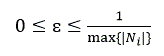(8)

The expression max{|Ni|} labels the number of the adjacent neighbors of the best connected entity.

### Experiments

In this paper, we examined how the topology of a distributed system affected the features of average consensus algorithm. We chose four topologies with the same size of 32 elements. The systems are of a tree (Fig.1), ring (Fig.2), star (Fig.3) and mesh topology. We changed three parameters: ε - the parameter determining the speed of the algorithm convergence (which also determines the interval of the convergence) and examined how this parameter affected il. As we assumed that the systems processing numbers of higher values suffered a precision loss of depicting decimal numbers, we calibrated the precision of the convergence event according to the initial values in all the experiments.

### The number of iterations necessary for achieving the consensus ε

In our first experiment, we examined il when all the parameters were set to the same values for all the topologies. We inserted the results of our simulations into the following table in order to compare the rate of the algorithm in these particular topologies together. We can see from the results in Tab.1 that the mesh topology, where all entities are each other's adjacent neighbour converged the fastest from all the topologies. The ring topology was the slowest, which was caused by the fact that it consisted of a lot of entities which were far from one another.Fig. 4 Results for the first experiment

### The impact of ε

In our second experiment, we changed the parameter ε and examined how these changes affected il. From the results shown in Fig.5, we can see that the function's values for the tree topology are decreasing as ε is increasing. For smaller ε, the function's growth is much significant than for larger ε. The function reaches its minimum approximately for ε = 0.37. From this point, the function rapidly grows for a narrow interval and then the system diverges.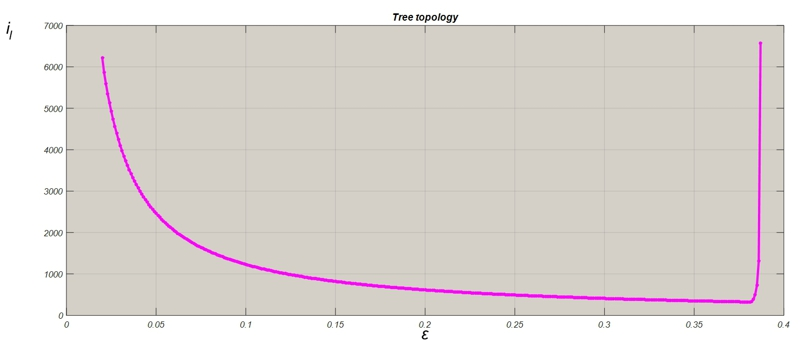Fig. 5 Impact of changes of ε- tree topologyl

The function's behaviour for ring topology is similar to the previous scenarios. The minimum is reached approximately for ε = 0,5.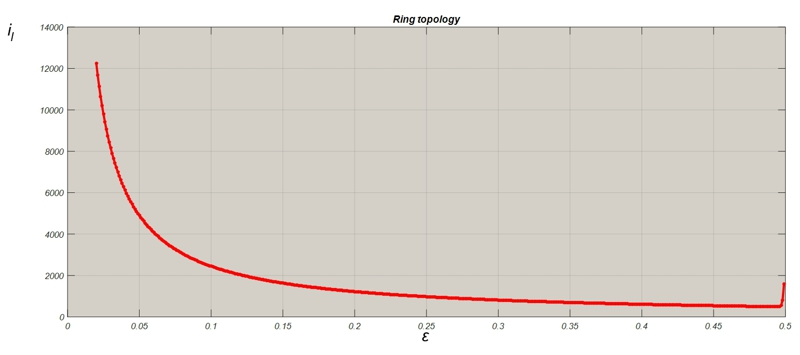Fig. 6 Impact of changes of ε - ring topology

The functions for the star and the mesh tology has similar behavior like the previous two. The minimums are reached approximately for ε = 0.06 (for the star topology) respectively ε = 0.03 (for the mesh topology).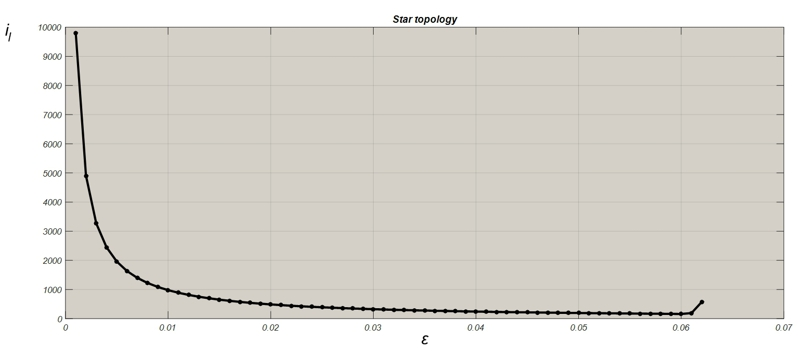Fig. 7 Impact of changes of ε- star topologyFig. 8 Impact of changes of ε - mesh topology

In Fig.9, we have shown the results gained from all the topologies. As we can see, the ring topology is the slowest within the examined topologies in this paper. It is caused by the fact that this topology has the lowest average connectivity. On the other hand, its interval of the convergence is the widest, which is caused by the fact that the best-connected entity has the smallest connectivity compared with other best-connected entities from the other topologies. The behaviour of the tree topology is similar to the ring topology's. In the average, it is better connected and also the best-connected entity has more adjacent neighbors; therefore, the topology is faster and the interval of the convergence is a bit narrower. The star topology requires a significantly smaller number of the iterations for reaching the consensus than the previous two. The interval of the convergence is narrow because of a strong connectivity of the best connected entity. It requires a large number of the iterations just for a small ε. The mesh topology is the fastest and requests a small number of the iterations for reaching the consensus also for small values of EPSI. As the connectivity of the best connected entities in the mesh and the star are equaled to each other, the interval of the convergence is almost the same. In the mesh topology, we can observe the phenomena that the interval of the convergence is symmetric according to the minimum.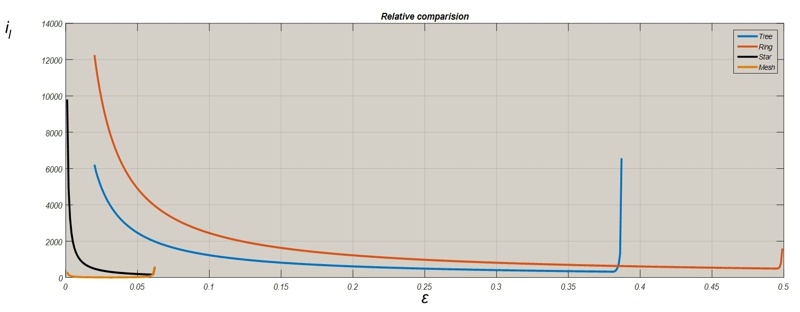Fig. 9 The relative comparision of the results from the second experiment

### Contribution

This paper deals with the impact of attributes of systems' topologies on the features of average consensus. We performed two experiments on four well-known topologies: tree, ring, star and mesh. We compared the number of iterations which are required for the systems to achieve the consensus when ε changed. From the obtained results, we can see that the better connected topologies are faster, but their interval of the convergence is narrower.

### Acknowledgment

Research described in this paper was financed by the National Sustainability Program under grant LO1401. For the research, infrastructure of the SIX Center was used.

### Literature

 Kenyeres, M., Kenyeres, J., Škorpil, V.: Effect of the speed of the algorithm's convergence on the quality of distributed computing in WSN Access server on-line. Praha 2015. http://access.feld.cvut.cz/view.php?nazevclanku=effect-of-the-speed-of-the-algorithms-convergence-on-the-quality-of-distributed-computing-in-wsn&cisloclanku=2015040001
 Abramowitz, Milton, and Irene A. Stegun, eds. Handbook of mathematical functions: with formulas, graphs, and mathematical tables. No. 55. Courier Dover Publications, 1972.
 Gibbons, Alan. Algorithmic graph theory. Cambridge UniversityPress, 1985.
 Bapat, Ravindra B. Graphs and matrices. Springer, 2010
 Glisic, Savo G., and Pentti A. Leppanen. Wireless communications: TDMA versus CDMA. Kluwer Academic Publishers, 1997.
 Biggs, Norman. Algebraic graph theory. Cambridge university press, 1993.
 Kenyeres, J., Kenyeres, M., Rupp, M., & Farkas, P. (2013). Connectivity-Based Self-Localization in WSNs. Radioengineering, 22(3).
 J. Kenyeres, M. Rupp, M. Kenyeres, and P. Farkaš, “WSN implementation of the average consensus algorithm,” in Proc. of 17th European Wireless Conference, Vienna, Austria, Apr. 27-29, 2011.